# 3. Compute () and (2) for the ground state of the harmonic oscillator potential (you will have to...3. Compute () and (2) for the ground state of the harmonic oscillator potential (you will have to look up the form of the wavefunction).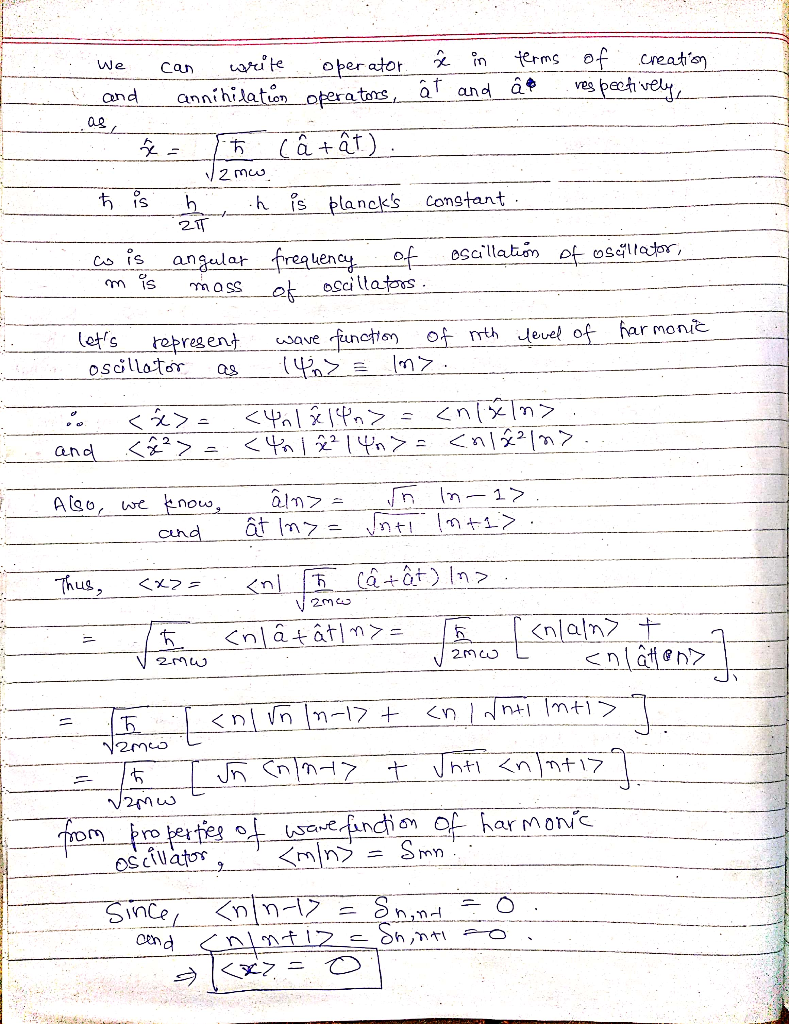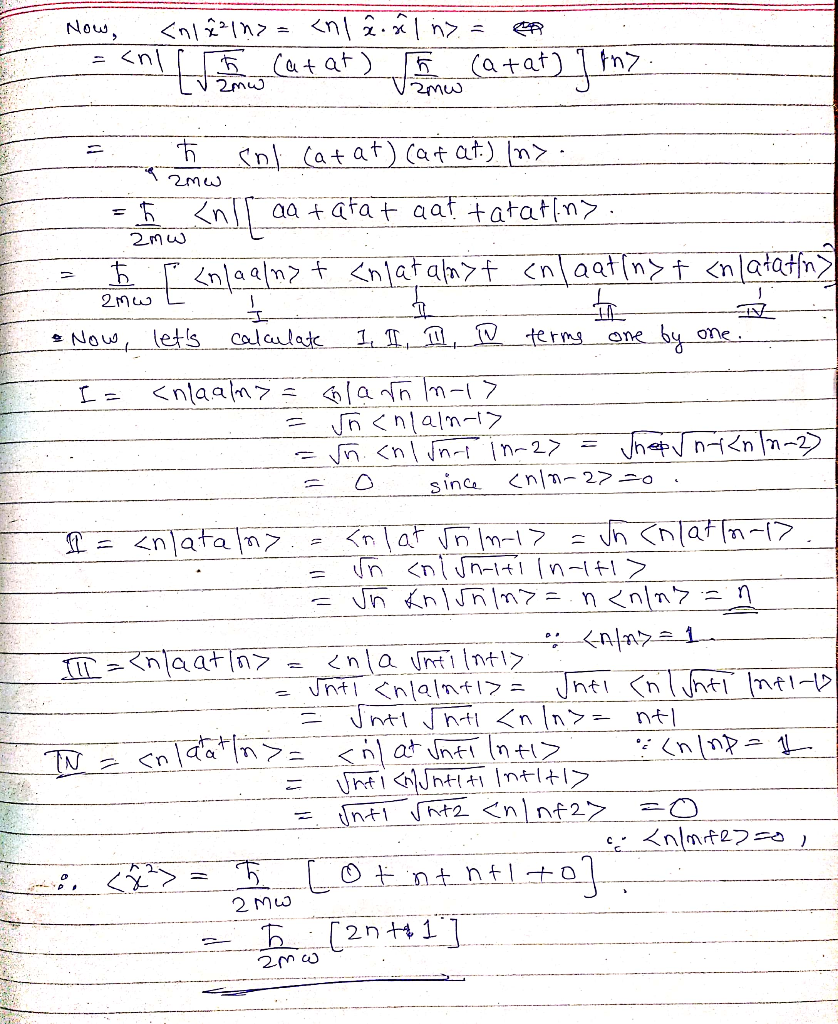##### Add Answer of: 3. Compute () and (2) for the ground state of the harmonic oscillator potential (you will have to...
Similar Homework Help Questions
• ### Spherically Symmetric Harmonic Oscillator Potential (QM)

A particle of mass m is in a three-dimension, spherically-symmetric harmonic oscillator potential given by V(r) = (1/2)Kr2. The particle is in the l = 0state. Find the ground-state radial wavefunction R(r) and the ground state energy.

• ### Ground State Harmonic Oscillator (Need by Monday night)

Confirm that the wavefunction for the ground state of aone-dimensional harmonic oscillator(a) a solution of the Schrodinger equation(b) that its energy is 1.2hbarωI think I have this figured out, but I'm not sure I did itright.

• ### quantum harmonic oscillatorVerify that the ground state (n=0) wavefunction is an eigenstate of the harmonicoscillator Hamiltonian. Using the explicit wavefunction of the ground state to calculatethe average potential energyand average kinetic energy . (Hints: takeadvantage of the symmetry in integration and use the formula:

• ### As a result of a sudden perturbation of the harmonic oscillator originally in the ground state, t...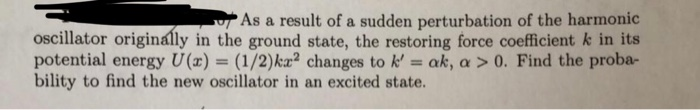As a result of a sudden perturbation of the harmonic oscillator originally in the ground state, the restoring force coefficient k in its potential energy U(a) (1/2)k2 changes to k' ak, a>0. Find the proba- bility to find the new oscillator in an excited state. As a result of a sudden perturbation of the harmonic oscillator originally in the ground state, the restoring force coefficient k in its potential energy U(a) (1/2)k2 changes to k' ak, a>0. Find the proba-...

• ### 2. Variational method. We can approximate the true ground-state wavefunction of the harmonic -프sxs and w(x) =D0 cos(cx...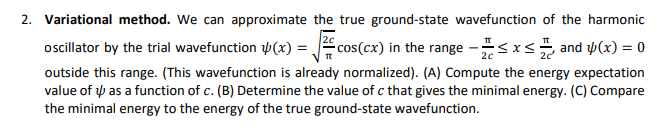2. Variational method. We can approximate the true ground-state wavefunction of the harmonic -프sxs and w(x) =D0 cos(cx) in the range oscillator by the trial wavefunction p(x) = X 2c 2c outside this range. (This wavefunction is already normalized). (A) Compute the energy expectation value of b as a function of c. (B) Determine the value of c that gives the minimal energy. (C) Compare the minimal energy to the energy of the true ground-state wavefunction 2. Variational method. We...

• ### What are and in the ground state of the harmonic oscillator?The Hamiltonian for two vibrating particles of masses m1 and m2 is given by: What are <x2> and <p2> in the ground state of the harmonic oscillator?

• ### For the ground state of a quantum harmonic oscillator, given by ?_0 = (?^(-1/2)*?^(-1/4))*?^(-(x^2)/(2?^2)) , show...

For the ground state of a quantum harmonic oscillator, given by ?_0 = (?^(-1/2)*?^(-1/4))*?^(-(x^2)/(2?^2)) , show that the expectation values for potential and kinetic energy are equal.

• ### 4. What is the extent of the ground state wavefunction of the vibration (approxi- mated as a harmonic oscillator)for NO. Note that vibrational transitions in NO are observed at 5.6 × 1013 Hz and its...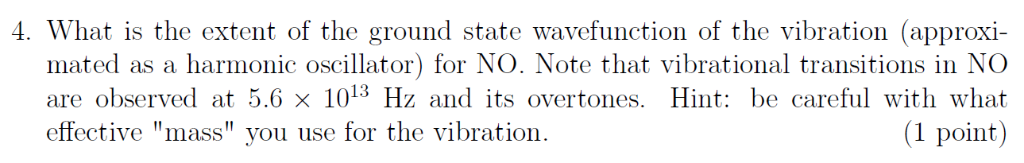4. What is the extent of the ground state wavefunction of the vibration (approxi- mated as a harmonic oscillator)for NO. Note that vibrational transitions in NO are observed at 5.6 × 1013 Hz and its overtones. Hint: be careful with what (1 point) effective "mass" vou use for the vibration. 4. What is the extent of the ground state wavefunction of the vibration (approxi- mated as a harmonic oscillator)for NO. Note that vibrational transitions in NO are observed at 5.6...

• ### Quantum Harmonic OscilatorAssume the form ?=Ne^(-ax^2) for the ground-state wavefunction of the harmonic oscillator, and substitute this into the schrodinger equatoin. Find the value of a thatmakes this an eigenfunction.problem 9.52 in book. http://i48.tinypic.com/ftm3pi.jpg?= greek letter psi for wavefunction

• ### The variational method can be used to solve for the ground state wavefunction and energy of a harmonic oscillator. Using a trail wavefunction of , where the function is defined between . The Hamilto...

The variational method can be used to solve for the ground state wavefunction and energy of a harmonic oscillator. Using a trail wavefunction of , where the function is defined between . The Hamiltonian operator for a 1D harmonic oscillator is Solving for the wavefunction gives Find that gives the lowest energy and compare from the trial function to the exact value, where coS We were unable to transcribe this imageWe were unable to transcribe this imageWe were unable to...

Need Online Homework Help?